首页 > 酒店 > 南京市> 南京香格里拉大酒店

### 南京香格里拉大酒店1,139

1.0分 /（249人评价）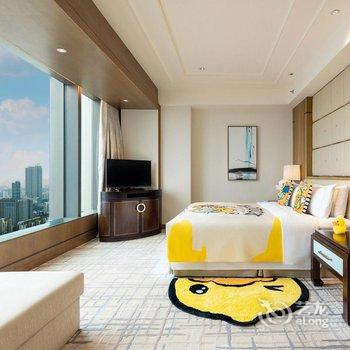•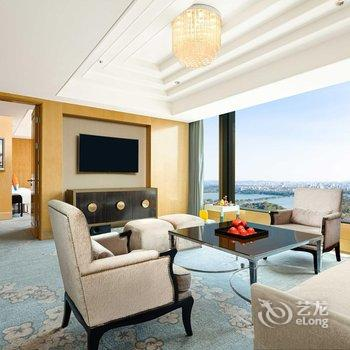••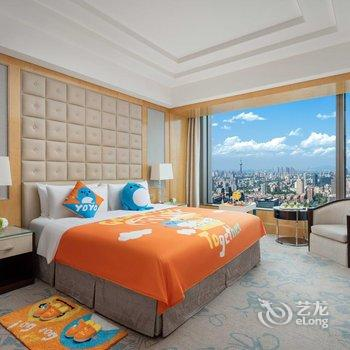•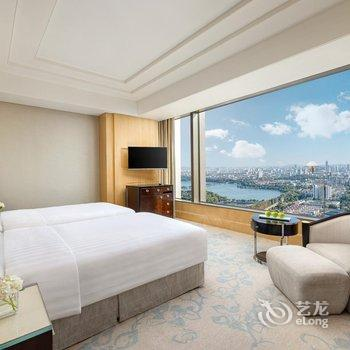•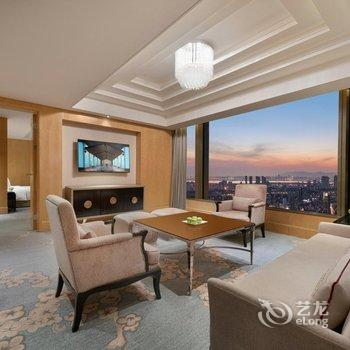•#### 豪华大床房

• 不含早（内宾）
• 大床2米
• 无窗
• 收费无线
• ￥1,139
• 有房
• 预付
• 预订
• 不含早（内宾）
• 大床2米
• 无窗
• 收费无线
• ￥1,175
• 有房
• 预付
• 预订
• 标准价
• 大床2米
• 无窗
• 收费无线
• ￥1,200
• 有房
• 担保
• 预订
• 标准价
• 大床2米
• 无窗
• 收费无线
• ￥1,283
• 有房
• 预付
• 预订
• 含双早（内宾）
• 大床2米
• 无窗
• 收费无线
• ￥1,334
• 有房
• 预付
• 预订
• 含单早（内宾）
• 大床2米
• 无窗
• 收费无线
• ￥1,334
• 有房
• 预付
• 预订
• 标准价
• 大床2米
• 无窗
• 收费无线
• ￥1,423
• 有房
• 担保
• 预订
• 标准价
• 大床2米
• 无窗
• 收费无线
• ￥1,423
• 有房
• 预付
• 预订
• 标准价
• 大床2米
• 无窗
• 收费无线
• ￥2,029
• 有房
• 担保
• 预订
• 标准价
• 大床2米
• 无窗
• 收费无线
• ￥1,819
• 满房
• 担保
• 满房#### 豪华双床房

• 标准价
• 双床1.2米
• 无窗
• 收费无线
• ￥1,443
• 有房
• 担保
• 预订
• 标准价
• 双床1.2米
• 无窗
• 收费无线
• ￥1,691
• 有房
• 预付
• 预订
• 不含早（预付）
• 双床1.2米
• 无窗
• 收费无线
• ￥1,812
• 有房
• 预付
• 预订
• 标准价
• 双床1.2米
• 无窗
• 收费无线
• ￥1,831
• 有房
• 担保
• 预订
• 标准价
• 双床1.2米
• 无窗
• 收费无线
• ￥1,831
• 有房
• 预付
• 预订
• 标准价
• 双床1.2米
• 无窗
• 收费无线
• ￥1,900
• 有房
• 预付
• 预订
• 标准价
• 双床1.2米
• 无窗
• 收费无线
• ￥2,021
• 有房
• 担保
• 预订
• 标准价
• 双床1.2米
• 无窗
• 收费无线
• ￥2,057
• 有房
• 预付
• 预订
• 标准价
• 双床1.2米
• 无窗
• 收费无线
• ￥2,349
• 满房
• 担保
• 满房#### 豪华湖景双床房

• 不含早（内宾）
• 双床1.2米
• 无窗
• 收费无线
• ￥1,726
• 有房
• 预付
• 预订
• 不含早（内宾）
• 双床1.2米
• 无窗
• 收费无线
• ￥1,782
• 有房
• 预付
• 预订
• 含单早（内宾）
• 双床1.2米
• 无窗
• 收费无线
• ￥1,892
• 有房
• 预付
• 预订
• 含双早（内宾）
• 双床1.2米
• 无窗
• 收费无线
• ￥1,925
• 有房
• 预付
• 预订
• 含单早（预付）
• 双床1.2米
• 无窗
• 收费无线
• ￥1,952
• 有房
• 预付
• 预订
• 标准价
• 双床1.2米
• 无窗
• 收费无线
• ￥2,433
• 满房
• 担保
• 满房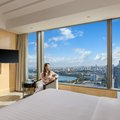#### 豪华湖景大床房

• 不含早（内宾）
• 大床2米
• 无窗
• 收费无线
• ￥1,795
• 有房
• 预付
• 预订
• 不含早（内宾）
• 大床2米
• 无窗
• 收费无线
• ￥1,853
• 有房
• 预付
• 预订
• 不含早（预付）
• 大床2米
• 无窗
• 收费无线
• ￥1,880
• 有房
• 预付
• 预订
• 含双早（内宾）
• 大床2米
• 无窗
• 收费无线
• ￥2,029
• 有房
• 预付
• 预订
• 含单早（内宾）
• 大床2米
• 无窗
• 收费无线
• ￥2,029
• 有房
• 预付
• 预订
• 标准价
• 大床2米
• 无窗
• 收费无线
• ￥2,201
• 有房
• 预付
• 预订
• 标准价
• 大床2米
• 无窗
• 收费无线
• ￥2,219
• 满房
• 担保
• 满房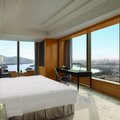#### 豪华阁豪华湖景大床房

• 含单早（内宾）
• 大床2米
• 无窗
• 收费无线
• ￥2,072
• 有房
• 预付
• 预订
• 含单早（内宾）
• 大床2米
• 无窗
• 收费无线
• ￥2,139
• 有房
• 预付
• 预订
• 标准价
• 大床2米
• 无窗
• 收费无线
• ￥2,176
• 有房
• 担保
• 预订
• 含双早（内宾）
• 大床2米
• 无窗
• 收费无线
• ￥2,257
• 有房
• 预付
• 预订
• 标准价
• 大床2米
• 无窗
• 收费无线
• ￥2,358
• 有房
• 预付
• 预订
• 标准价
• 大床2米
• 无窗
• 收费无线
• ￥2,794
• 有房
• 预付
• 预订
• 标准价
• 大床2米
• 无窗
• 收费无线
• ￥2,520
• 有房
• 预付
• 预订
• 标准价
• 大床2米
• 无窗
• 收费无线
• ￥2,702
• 满房
• 担保
• 满房#### 豪华阁尊荣湖景大床房

• 含单早（内宾）
• 大床2米
• 无窗
• 收费无线
• ￥2,244
• 有房
• 预付
• 预订
• 标准价
• 大床2米
• 无窗
• 收费无线
• ￥2,274
• 有房
• 预付
• 预订
• 标准价
• 大床2米
• 无窗
• 收费无线
• ￥2,274
• 有房
• 担保
• 预订
• 标准价
• 大床2米
• 无窗
• 收费无线
• ￥2,390
• 有房
• 预付
• 预订
• 标准价
• 大床2米
• 无窗
• 收费无线
• ￥2,390
• 有房
• 担保
• 预订
• 标准价
• 大床2米
• 无窗
• 收费无线
• ￥2,510
• 有房
• 担保
• 预订
• 标准价
• 大床2米
• 无窗
• 收费无线
• ￥3,024
• 有房
• 预付
• 预订#### 豪华阁行政湖景套房

• 标准价
• 大床2米
• 无窗
• 收费无线
• ￥2,915
• 有房
• 预付
• 预订
• 标准价
• 大床2米
• 无窗
• 收费无线
• ￥2,915
• 有房
• 担保
• 预订#### 270度全湖景特色套房

• 标准价
• 大床2米
• 无窗
• 收费无线
• ￥8,279
• 有房
• 担保
• 预订
• 标准价
• 大床2米
• 无窗
• 收费无线
• ￥9,302
• 有房
• 预付
• 预订

• 上网服务
免费wifi
• 酒店电话
+86-25-86308888
• 停车场
免费停车场
• 开业时间
酒店开业时间 2014-10-01 新近装修时间
• 酒店设施